# MeshSphere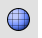Mesh

Polygon Mesh Primitives >

Sphere

The MeshSphere command draws a polygon mesh sphere.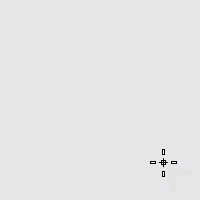Command-line options

Creates a sphere by picking the center point and a radius point.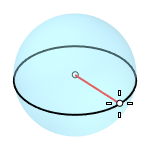### 2Point

Creates a sphere from two opposite points on the base circle.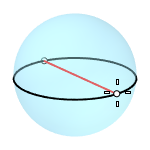### 3Point

Creates a sphere from three points on the base circle.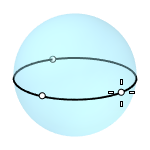### Tangent

Creates a sphere with the base circle tangent to one, two or three curves.### AroundCurve

Creates a sphere from its center point on a curve, and a point on the base circle perpendicular to the curve.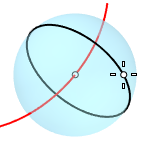### 4Point

Creates a sphere from three points on a section circle and a point on the sphere.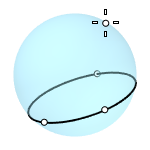### FitPoints

Creates a sphere by fitting to selected point objects, curve and surface control points, and mesh vertices.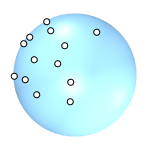### VerticalFaces

The face count in the vertical direction.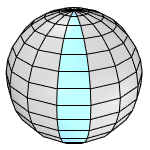### AroundFaces

The face count in the horizontal direction.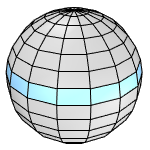#####Sphere

Draw a solid sphere.

#####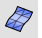Mesh

Create a mesh from a NURBS surface or polysurface.

Rhinoceros 6 © 2010-2020 Robert McNeel & Associates. 29-Jul-2020